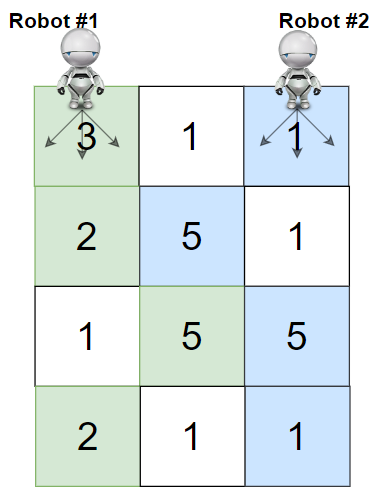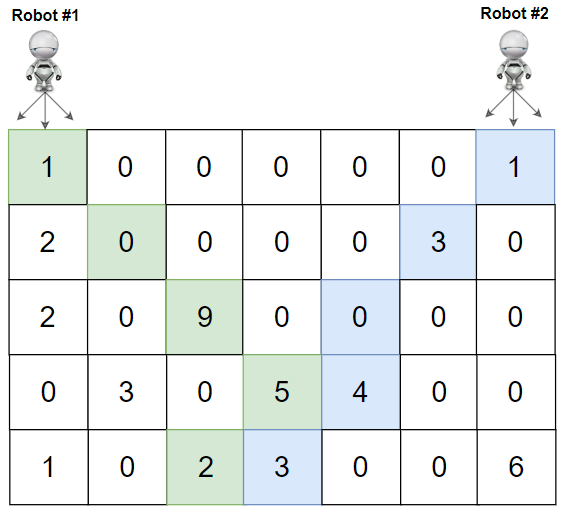# 1463. Cherry Pickup II

January 07, 2022

## Description:

You are given a rows x cols matrix grid representing a field of cherries where grid[i][j] represents the number of cherries that you can collect from the (i, j) cell.

You have two robots that can collect cherries for you:

• Robot #1 is located at the top-left corner (0, 0), and
• Robot #2 is located at the top-right corner (0, cols - 1).

Return the maximum number of cherries collection using both robots by following the rules below:

• From a cell (i, j), robots can move to cell (i + 1, j - 1), (i + 1, j), or (i + 1, j + 1).
• When any robot passes through a cell, It picks up all cherries, and the cell becomes an empty cell.
• When both robots stay in the same cell, only one takes the cherries.
• Both robots cannot move outside of the grid at any moment.
• Both robots should reach the bottom row in grid.

### Example 1:### Example 2:### Constraints:

• rows == grid.length
• cols == grid[i].length
• 2 <= rows, cols <= 70
• 0 <= grid[i][j] <= 100

We have a maximum input size of 70*70 = 4900, a loose limit would be $O((mn)^2)$.

### Intuition:

• Each round, each robot would go exactly one step down. And they will always move forward until reaching the end.
• In this way, we can describe the status of two robots by a 3-element tuple (y,x1,x2). Since #y: row #x: col, there are $O(r*c^2)$ combinations
• dp[y][x1][x2] means the maximum cherries collected at this combination
• Base Case: y>=r, x<0 or x>c (out of bounary), return 0
• Transition Equation: dp[y+1][x1][x2] ={max[y][x1'][x2'] + grid[y][x1]+grid[y][x2] if x1!=x2 else 0}
• Time Complexity: $O(rc^29)$
• Space Complexity: $O(r*c^2)$
• To get the answer: find the maximum value in dp[r-1][x1][x2] (traverse)

### A Reverse Solution:

Robots start at any grid in the last level, and then they go up until one goes to the left corner, the other go to the right corner.

• Transition Equation: dp[y][x1][x2] ={max[y+1][x1'][x2'] + grid[y][x1]+grid[y][x2] if x1!=x2 else 0}

• To get the answer: dp[c-1]